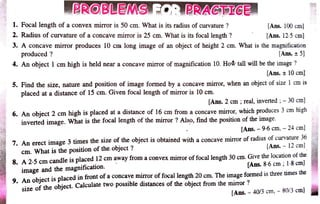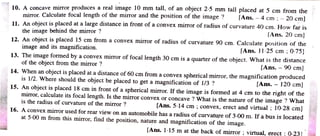Publicité1 sur 2
Publicité

### Light Reflection.pdf

1. PROBLEMS.FOR PRACTICE 1. Focal length of a convex mirror is 50 cm. What is its radius of curvature ? [Ans. 100 cm] 2. Radius of curvature of a concave mirror is 25 cm. What is its focal length ? 3. A concave mirror produces 10 ca long image of an object of height 2 cm. What is the magnification produced ? [Ans. 12-5 cm] [Ans.+5] 4. An object 1 cm high is held near a concave miror of magnification 10. How tall will be the image ? [Ans. 10cm] 5. Find the size, nature and position of image formed by a concave mirror, when an object of size 1 cmis placed at a distance of 15 cm. Given focal length of mirror is 10 cm. [Ans. 2 cm ; real, inverted; - 30 cm] 6. An object 2 cm high is placed at a distance of 16 cm from a concave mirror, which produces 3 cm high inverted image. What is the focal length of the miror ? Also, find the position of the image. [Ans. - 9-6 cm, - 24 cm] 7. An erect image 3 times the size of the object is obtainèd with a concave mirror of radius of curvature 36 cm. What is the position of the.object ? 8. A 2.5 cm candle is placed 12 cm away from a convex mirror of focal length 30 cm. Give the location of the image and the magnification. 9. An object is placed in front of a concave mirror of focal length 20 cm. The image formed is three times the size of the object. Calculate two possible distances of the object from the mirror ? [Ans. 12 cm] [Ans. 8-6 cm; 1-8cm] [Ans. 40/3 cm, - 80/3 cm]
2. 10. A concave mirror produces a real image 10 mm tall, of an object 2-5 mm tall placed at 5 cm from the mirror. Calculate focal length of the mirror and the position of the image ? 11. An object is placed at a large distance in front of a convex miror of radius of curvature 40 cm. How far is the image behind the mirror ? [Ans. 4 cm;-20cm] TAns. 20 cm] 12. An object is placed 15 cm from a convex mirror of radius of curvature 90 cm. Calculate position of the image and its magnification. Ans. 1125 cm 0-75] 13. The image formed by a convex mirror of focal length 30 cm is a quarter of the object. What is the distance of the object from the mirror? 14. When an object is placed at a distance of 60 cm from a convex spherical mirror, the magnification produced is 1/2. Where should the object be placed to get a magnification of 1/3 ? 15. An object is placed 18 cm in front of a spherical mirror. If the image is formed at 4 cm to the right of the mirror, calculate its focal length. Is the mirror convex or concave ? What is the nature of the image ? What is the radius of curvature of the mirror ? [Ans. 90cm] [Ans. 120cm] [Ans. 5.14 cm; convex, erectand virtual; 10-28 cn] 16. A convex mirror used for rear view on an automobile has a radius of curvature of 3-00 m. If a bus is located at 5-00 m from this mirror, find the position, nature and magnification of the image. [Ans. 1.15 m at the back of mirror ; virtual, erect; 0-231
Publicité